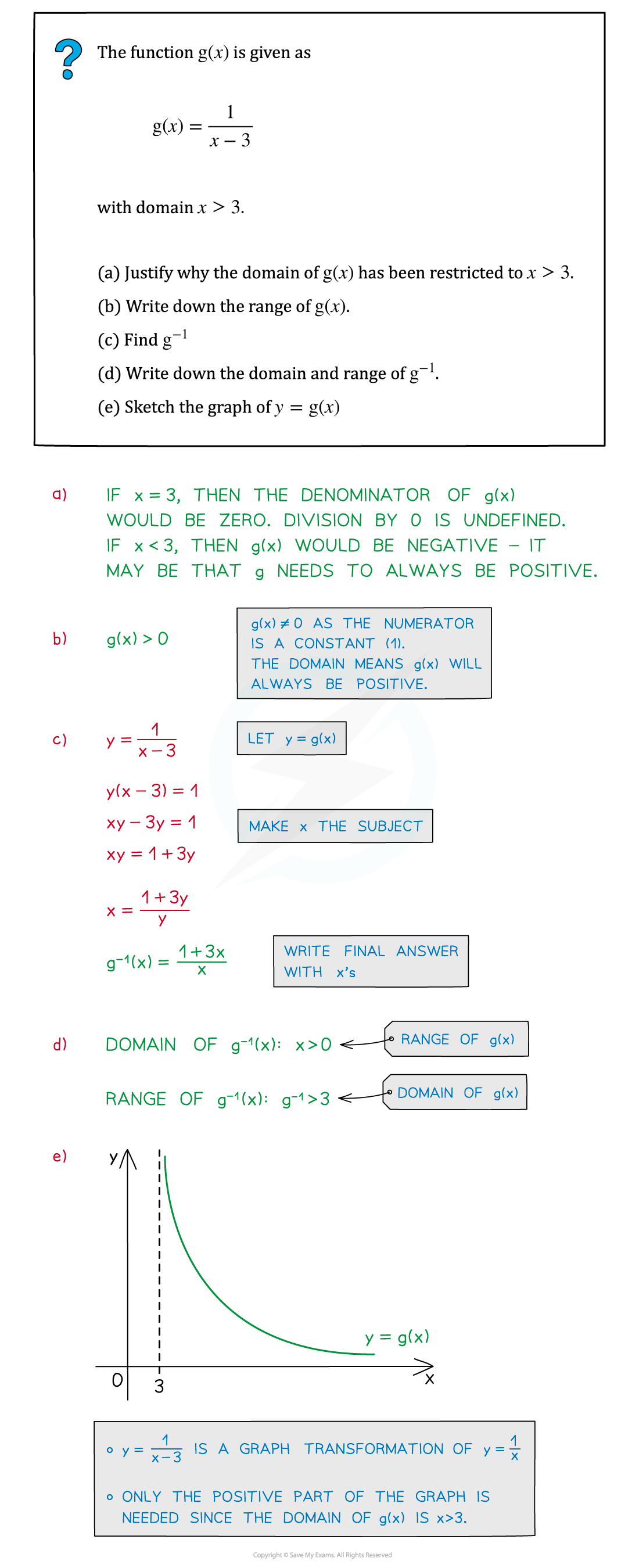# Edexcel IGCSE Maths 复习笔记 3.2.4 Functions - Domain, Range & Exclusions

Edexcel IGCSE Maths 复习笔记 3.2.4 Functions - Domain, Range & Exclusions

#### What are functions?

• Functions are a formal way of writing mathematical expressions
• eg. f(x) = 3x + 2 would be a linear function
• eg2. g : x ⟼ x2 + 3x – 5 would be a quadratic function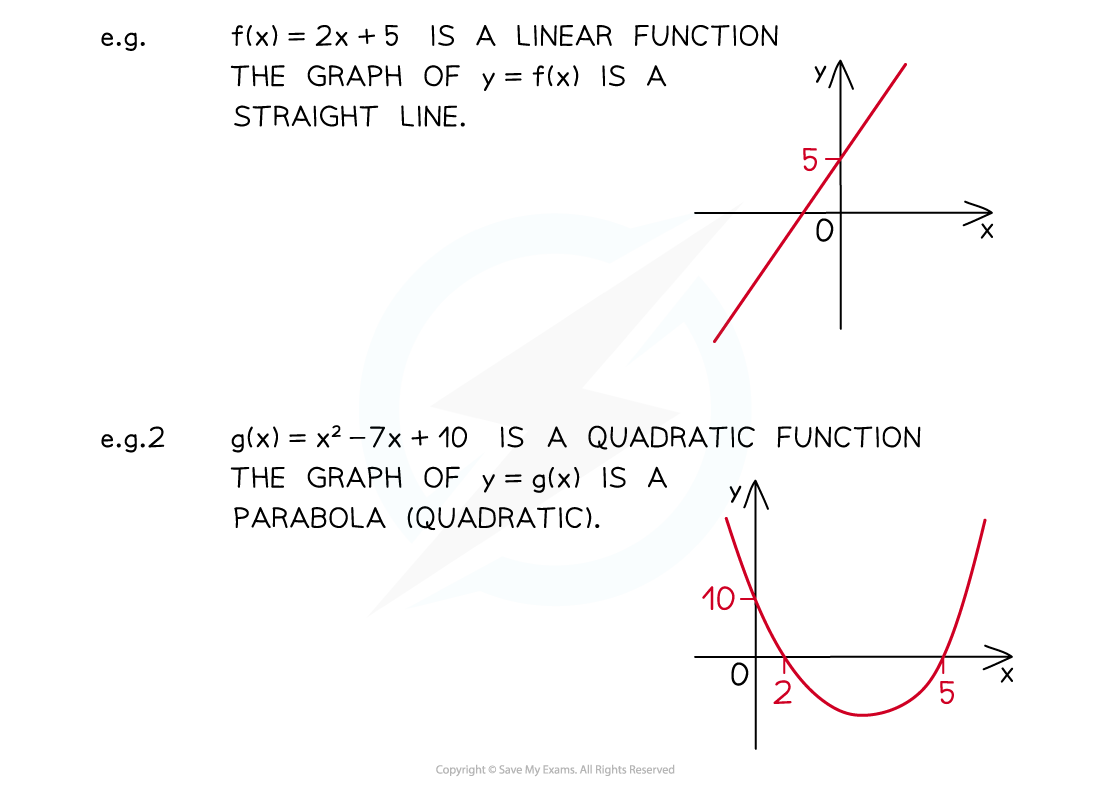#### What is the domain of a function?

• The domain of a function is the values of x (the “input”) are allowed to take
• For some functions, x cannot be certain values
• eg. f(x) = 1 / xx cannot take the value 0

• Other times we may choose to restrict the values of x
• eg. The function g(x) = 5 – 2x2 is used to model the height of water throughout the day where x indicates timeIt may make sense to limit x so it only covers a 24 hour period

• Inequalities are used to describe the values x can take
• Any exclusions are usually indicated using the “not equal to” symbol ()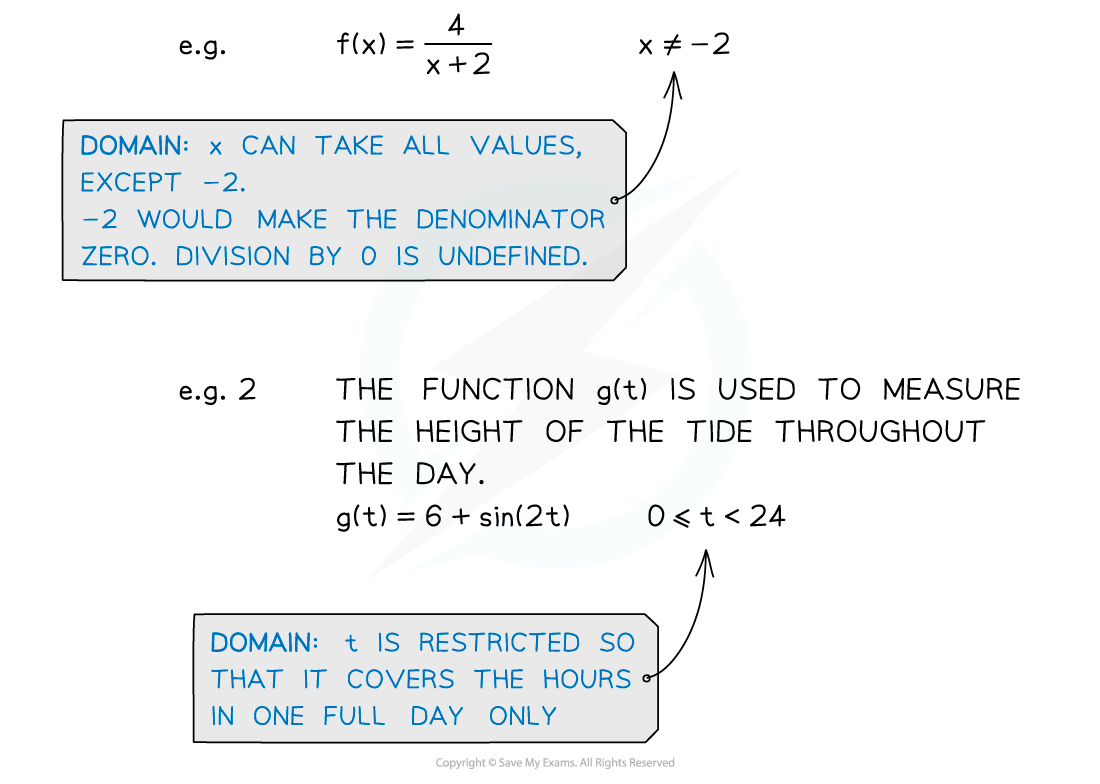#### What is the range of a function?

• The range of a function is the values of f (the “output”) that could occur
• Some functions can never take certain values, regardless of the value of x
• eg. f(x) = x2f, a squared (real) value, cannot be negative
• eg2. g(x) = 1 / xg can never be zero (because numerator cannot be 0)

• The range of a function can be influenced by its domain
• As with the domain, inequalities are used to describe the values a function can take and “not equal to” () is used for any exclusions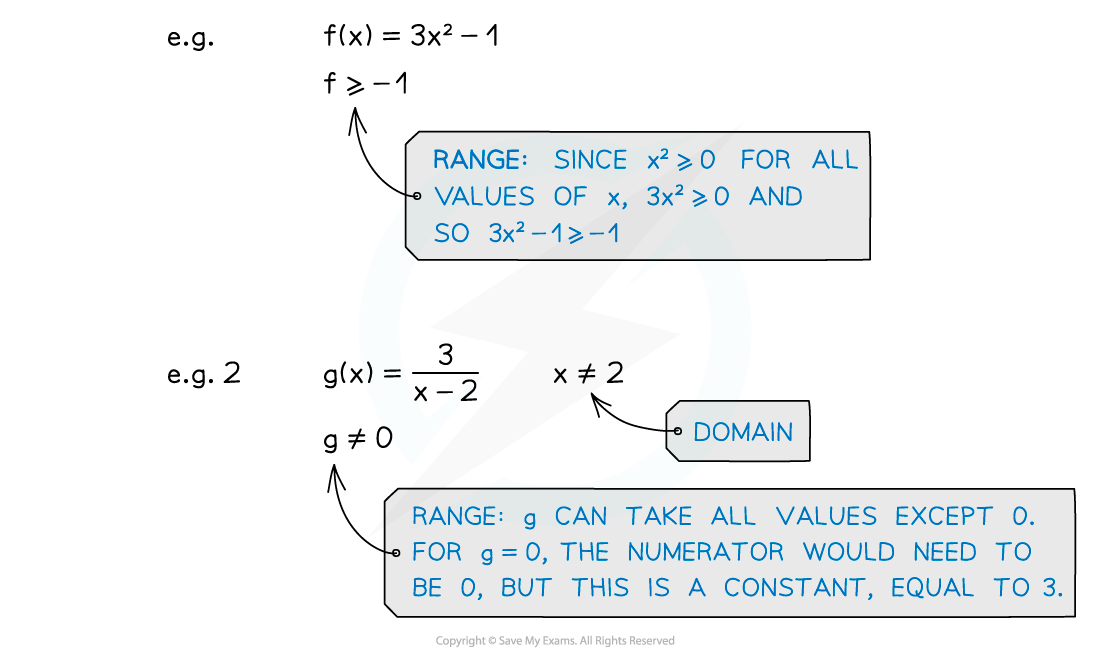#### How do I solve problems involving the domain and range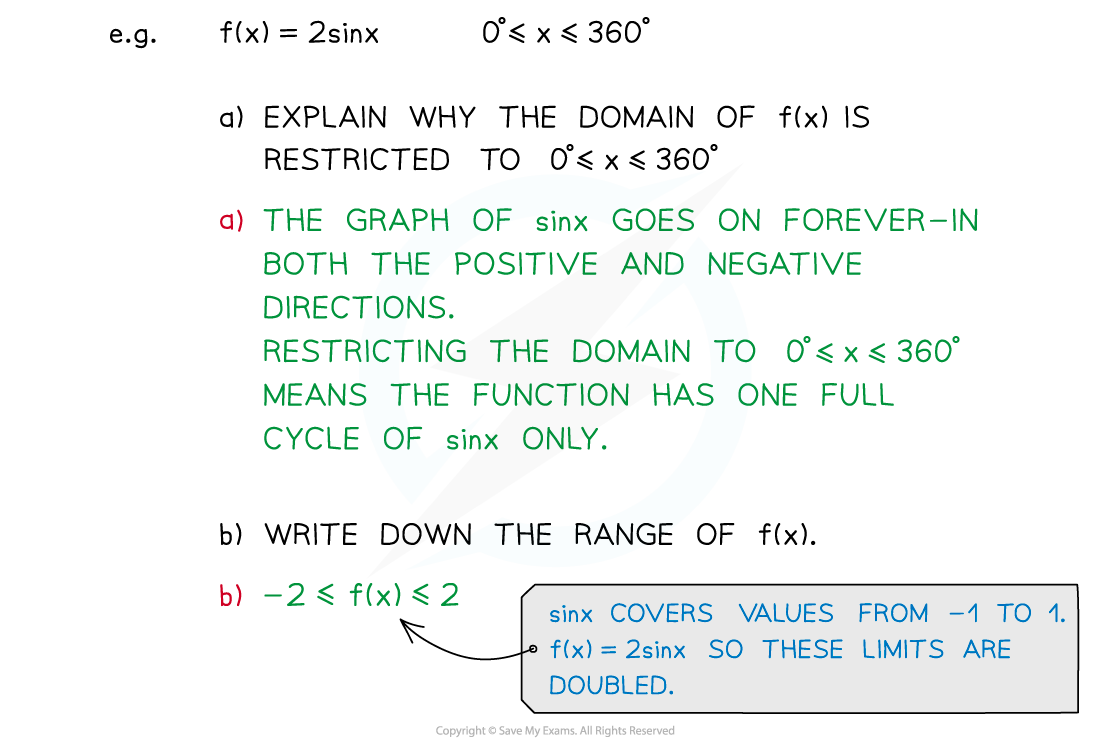• You need to be able to identify and explain any exclusions in the domain of a function
• You need to be able to deduce the range of a function from its expression and domain
• You may also be asked to sketch a graph of a function
• This could involve sketching parts of familiar graphs that are restricted because of the domain and exclusions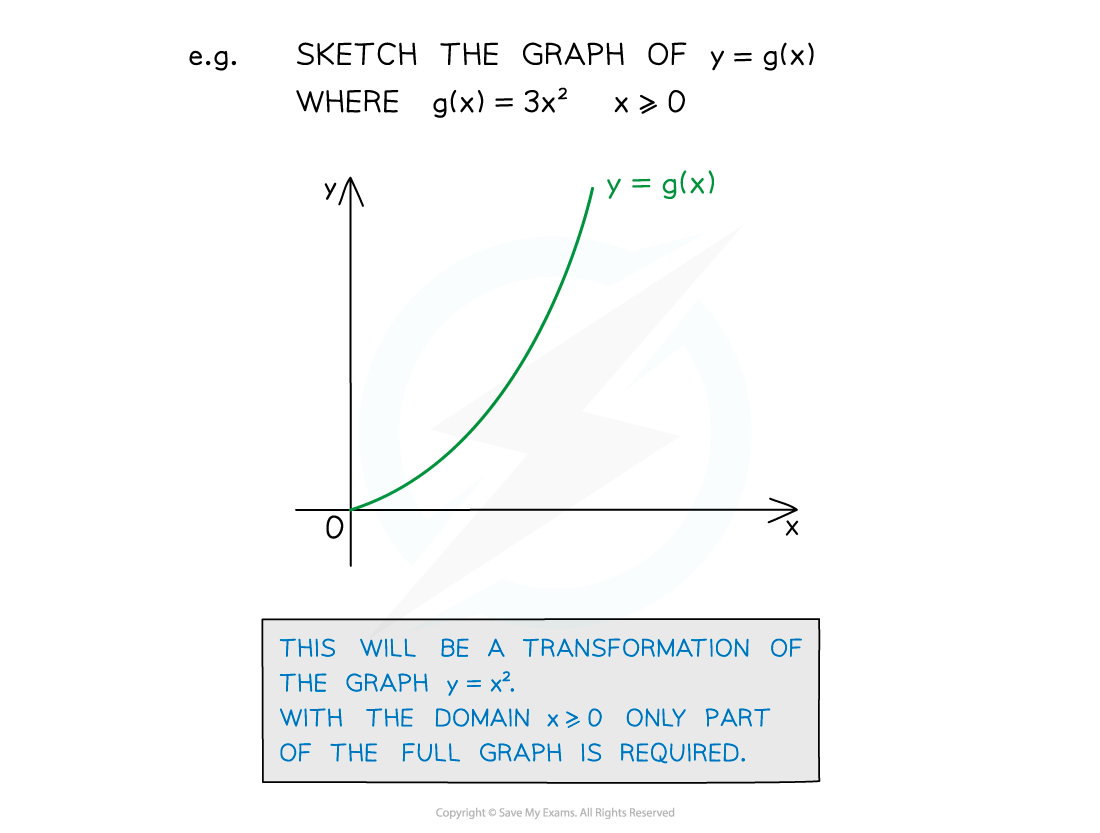#### Domain and range of inverse functions

• Make sure you are familiar with inverse functions, denoted by f-1g-1, etc.
• The range of a function is the domain of the inverse function
• The domain of a function is the range of the inverse function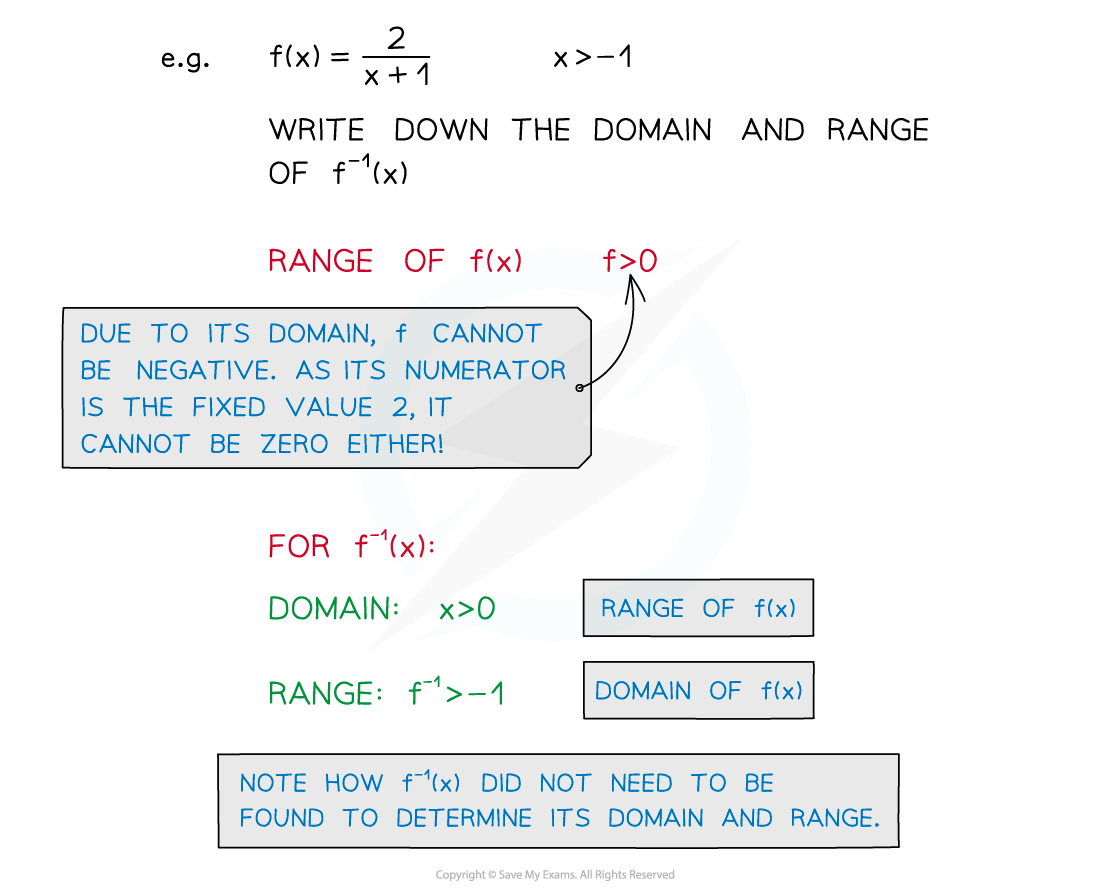#### Exam Tip

A graph of the function can help “see” both the domain and range of function, and a sketch can help if you have not been given a diagram.

#### Worked Example